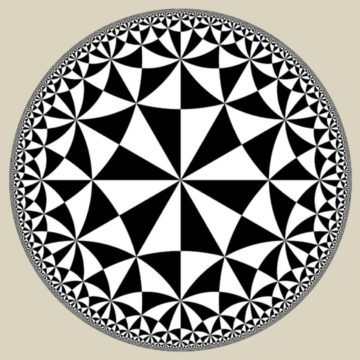# Theoretical physicist Sean Carroll visualizes a mind-bending geometry that inspired many, from Einstein to Escher

Sean Carroll in Pioneer Works:Of Euclid’s five postulates, which form the basis of Euclidean geometry, the fifth is the most controversial. Called the Parallel Postulate, it’s a pretty basic idea. Take a flat piece of paper, draw a line segment and then draw two lines that are perpendicular to it. The Parallel Postulate simply says that the lines will remain forever parallel, staying precisely the same distance apart even if we extend them to infinity on either side of the line segment.

For a long time mathematicians strived to prove the Parallel Postulate using the first four (more) basic postulates, and so elevate it from a postulated axiom to a proven theorem. It turns out that you can’t, and for good reason—the postulate isn’t true for all geometries. Euclidean geometry, represented by the flat piece of paper, and on which lines that start off parallel never intersect, is just one kind of geometry, but there are others. You can imagine replacing the Parallel Postulate with some alternative: maybe lines that are parallel initially draw closer together and touch eventually, or maybe they always move apart. The first option is the case with spherical geometry, which describes shapes drawn on the surface of a sphere (think of lines of longitude that start of parallel at the equator but converge at the poles), while the second defines hyperbolic geometry, which is more like the surface of a saddle or a Pringle’s potato chip.

These non-Euclidean alternatives weren’t noticed for millennia. Euclid himself flourished around 300 BCE, and the first alternatives to the Parallel Postulate were proposed in the early 1800s by Russian mathematician Nikolai Lobachevsky and Hungarian mathematician János Bolyai. Interestingly, both studied the hyperbolic alternative to flat Euclidean geometry.

This raises two questions: First, why did it take so long? And second, why did the mathematicians study hyperbolic geometry before spherical? Everyone knew about spheres. How hard could it have been to imagine drawing some lines on them, and exploring the geometric consequences of doing so?

The questions share a common answer.

More here.